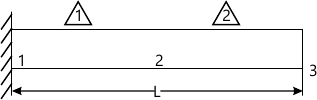MORE IN Finite Element Analysis
MU Mechanical Engineering (Semester 8)
Finite Element Analysis
December 2012
Total marks: --
Total time: --
INSTRUCTIONS
(1) Assume appropriate data and state your reasons
(2) Marks are given to the right of every question
(3) Draw neat diagrams wherever necessary

1(a)

The following differential equation is available for a physical phenomenon $$\dfrac{d^{2}u}{dx^{2}}+u+x=0$$

,$$0â‰¤ x â‰¤1$$, $$u(0)=u(1)=0$$. Solve above equation,using subdomain method and Galerkin method.

10 M
1(b)

Derive the cubic shape function of Largrange's family.what are the characteristic of the shape function? Plot the shape function along of the element. State the difference shape function and interpolation function?

10 M

2(a)

Explain the following:
1.Global Local and Natural Co-ordinate system
2.Boundary condition and its types.

10 M
2(b) For a uniform cross-section bar shown in Figure below of length 1 meter made up of a matherial having youngs modulus 2*1011 N/m2 and $$\rho=7800kg/m^3$$. Estimate the natural frequencies of axial vibration of the bar using both consistent and lumped mass matrices. Use a two element mesh. If the exact solition is given by the relation $$\omega_{i} =\frac{i\pi}{2L}\sqrt{\frac{E}{\rho}}$$ where $$i=1,2,3,4,5...$$10 M

3(a) Analyse the truss completely i.e for displacement,reaction tresses and strain given I fig.
Area of each member =1000mm2
E for each member=210 Gpa
P1=10 KN
P2=20kN.
14 M
3(b) Explain the basic units of a typical finite elements program.
6 M

4(a)

Evaluate the following integral $$I=\int_{0}^{4} x^{3}dx\\$$
Using (a) trapezoidal rule
(b) Simpsons one third rule
Compare the solution with the exact solution.

6 M
4(b) What are Eigen value problems? Explain any one algorithm associated with it.
14 M

5(a)

The governing differential equation for a rod loaded axial force is
$$\frac{d}{dx}(AE\frac{du}{dx})+q =0$$, Where E is Young's modulus of elasticity, A is the area of cross section. q is the load intensity and u is the axial displacement. Obtain the variational form for this equation
Assume that the boundary condition are
At $$x=0,u =0$$
at , $$x=l, EA \dfrac{du}{dx}=P$$
where $$l$$ is the length of the rod.

14 M
5(b) What are serendipity elements.Derive and graphically represents interpolation functions for 8 needed rectangular element.
14 M

6(a) Explain steps involved in solving time dependent problems.
6 M
6(b) Analysis completely the problem given,using directly the Element matrix equation corresponding to that field
==IMAGE===
All length and diameter are in mm.
14 M

Any four
7(a) Write short notes on (any four);
Generalised Jacobi method
5 M
7(b) Sources of error in FEM
5 M
7(c) Shape functions for triangular element
5 M
7(d) Minimum potential Energy principle
5 M
7(e) Isoparametric elements
5 M
7(f) h and μ method of FEM.
5 M

More question papers from Finite Element Analysis